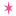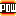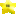Sprite DBBy Category
Welcome to the Sprite DB! Here you will find information on how to use any sprite in NSMB.
You can also have this database in NSMB Editor if you're using the latest version!

All the registered users can also collaborate with the Sprite DB by sharing their sprite data findings. Click any sprite below to edit it.

You need to be logged in to edit the sprite database.
All sprites

Unknown

Known, incomplete

Known, complete

Uncategorized
Platforms and ropes
Event controllers
Bosses and cutscenes
Camera controllers
Useless sprites
Effects and decorations
Other
Enemies - Overground
Enemies - Underground
Enemies - Water
Enemies - Snow
Enemies - Fire
Enemies - Castle
Enemies - Ghost House
Enemies - Desert

Go to sprite ID:
Status
326 total
292
32
2

The above bar needs more green. HELP US MAKE IT HAPPEN! NOW!
ID Class ID Name Last edited by
93 77Arrow signboard
 Direction: list at nybble 10: 0=Left,1=Left-down,2=Down,3=Right-down,4=Right,5=Right-up,6=Up,7=Left-up Mirrored: checkbox at nybble 11 with mask 1 Smaller: checkbox at nybble 7 with mask 1 Shift position: list at nybble 8-9: 0=No shifting,16=8 pixels right,1=8 pixels down,17=8 pixels right and downMeroMero (rev. 13)
96 301SeaweedRicBent (rev. 5)
231 278Water
 Trigger ID 1: value at nybble 2-3. On ID 2 the water will rise, but have to be activated again with a stop command to lower. Trigger ID 2: value at nybble 0-1. On ID 1 the water will rise then lower. (This is wrong, Hiccup thinks[E version]) Water lowering's delay: value at nybble 4. Any value other than 0 will override both Input IDs. Water rising's delay: value at nybble 5. Any value other than 0 will override both Input IDs. Opacity: value at nybble 6-7. Modulo 32. 0 is transparent. 31 has the highest opacity. Does not do anything or does not exist for Lava and Poison Water. Tiles to move: value at nybble 8-9 Speed: value at nybble 10-11. Modulo 128. If lower than 128 then the water will rise, if greater or equal than 128 the water will lower. Lowering water does not work properly. If you want lowering water, rise it with a enter-view event, then "unrise" it or get the code hack that fixes the problem.Hiccup (rev. 18)
234 279Lava
 Trigger ID 1: value at nybble 2-3 Trigger ID 2: value at nybble 0-1. Without either input ID, the lava will rise automatically. If you set a value for either one of the IDs by themselves, the lava will rise to the height you select when that ID is activated. ID2 will only make the lava rise, and will wait for an off command from the ID2 activator again. If you set a values for both IDs, ID1 will make the lava go up then down. Unknown 6: value at nybble 6. Nybbles 6 and 7 don't seem to have an effect. But they are used ingame. Nybbles 4 and 5 could still do something. Unknown 7: value at nybble 7 Tiles to rise: value at nybble 8-9 Speed to rise: value at nybble 10-11. Modulo 128. If lower than 128 then the lava will rise, if greater or equal than 128 the lava will lower.Hiccup (rev. 8)
255 280Jungle FG Effect (Unused Actor)
 Swimmable: checkbox at nybble 4-5 with mask Scroll Up/Down: signed value at nybble 10-11. If swimmable, the image doesn't move. If negative value it scrolls down, if positive value it scrolls up.Hiccup (rev. 8)
259 281Poisoned Water
 Trigger ID 1: value at nybble 2-3 Trigger ID 2: value at nybble 0-1 Unknown 6: value at nybble 6. Nybbles 6 and 7 don't seem to have an effect. But they are used ingame. Nybbles 4 and 5 could still do something. Unknown 7: value at nybble 7 Tiles to Rise: value at nybble 8-9 Speed: value at nybble 10-11. Modulo 128. If lower than 128 then the poisoned water will rise, if greater or equal than 128 the poisoned water will lower.MeroMero (rev. 10)
277 125Arrow sign
 Z order: list at nybble 4: 0=Model,1=Tileset,2=Foreground,3=Background. 0, 2 and 3 are unused. Direction: list at nybble 5: 0=Up,1=Up/right,2=Right,3=Down/right,4=Down,5=Down/left,6=Left,7=Up/leftHiccup (rev. 8)
310 287Fog FG effect
 Direction: list at nybble 4: 0=Left-down,1=Left-up,2=Right-down,3=Right-up Opacity: value at nybble 5-6. Modulo 32. 0 is transparent, 30 is almost opaque, 31 is completely red. Mixed shift: value at nybble 7. Shift horizontally and vertically. Horizontal scrolling: value at nybble 8. 1 is the slowest, 16 is the fastest. Vertical scrolling: value at nybble 9. 1 is the slowest, 16 is the fastest. Horizontal speed: value at nybble 10. 1 is the slowest, 16 is the fastest. Vertical speed: value at nybble 11. 1 is the slowest, 16 is the fastest.poudink (rev. 6)
311 291Snow FG effect 1
 Swaying start position: value at nybble 4. Also doubles as a direction value (Modulo 4) if nybble 10 and/or nybble 11 are not null. 0 is left-down, 1 is left-up, 2 is right-down and 3 is right-up. Swaying distance: value at nybble 5. 0 is static. Swaying speed: value at nybble 6. 0 is static, 15 is the fastest. Mixed shift: value at nybble 7. Shift horizontally and vertically. Horizontal scrolling: value at nybble 8. 1 is the slowest, 16 is the fastest. Vertical scrolling: value at nybble 9. 1 is the slowest, 16 is the fastest. Horizontal speed: value at nybble 10. 1 is the slowest, 16 is the fastest. Vertical speed: value at nybble 11. 1 is the slowest, 16 is the fastest.MeroMero (rev. 2)
313 292Snow FG effect 2
 Swaying start position: value at nybble 4. Also doubles as a direction value (Modulo 4) if nybble 10 and/or nybble 11 are not null. 0 is left-down, 1 is left-up, 2 is right-down and 3 is right-up. Swaying distance: value at nybble 5. 0 is static. Swaying speed: value at nybble 6. 0 is static, 15 is the fastest. Mixed shift: value at nybble 7. Shift horizontally and vertically. Horizontal scrolling: value at nybble 8. 1 is the slowest, 16 is the fastest. Vertical scrolling: value at nybble 9. 1 is the slowest, 16 is the fastest. Horizontal speed: value at nybble 10. 1 is the slowest, 16 is the fastest. Vertical speed: value at nybble 11. 1 is the slowest, 16 is the fastest.MeroMero (rev. 5)
314 293Snow FG effect 3
 Swaying start position: value at nybble 4. Also doubles as a direction value (Modulo 4) if nybble 10 and/or nybble 11 are not null. 0 is left-down, 1 is left-up, 2 is right-down and 3 is right-up. Swaying distance: value at nybble 5. 0 is static. Swaying speed: value at nybble 6. 0 is static, 15 is the fastest. Mixed shift: value at nybble 7. Shift horizontally and vertically. Horizontal scrolling: value at nybble 8. 1 is the slowest, 16 is the fastest. Vertical scrolling: value at nybble 9. 1 is the slowest, 16 is the fastest. Horizontal speed: value at nybble 10. 1 is the slowest, 16 is the fastest. Vertical speed: value at nybble 11. 1 is the slowest, 16 is the fastest.MeroMero (rev. 3)
315 288Cloud FG effect
 Direction: list at nybble 4: 0=Left-down,1=Left-up,2=Right-down,3=Right-up Opacity: value at nybble 5-6. Modulo 32. 0 is transparent, 30 is almost opaque, 31 is completely red. Mixed shift: value at nybble 7. Shift horizontally and vertically. Horizontal scrolling: value at nybble 8. 1 is the slowest, 16 is the fastest. Vertical scrolling: value at nybble 9. 1 is the slowest, 16 is the fastest. Horizontal speed: value at nybble 10. 1 is the slowest, 16 is the fastest. Vertical speed: value at nybble 11. 1 is the slowest, 16 is the fastest.poudink (rev. 10)
316 289Water FG effect 1
 Swaying start position: value at nybble 4. Also doubles as a direction value (Modulo 4) if nybble 10 and/or nybble 11 are not null. 0 is left-down, 1 is left-up, 2 is right-down and 3 is right-up. Swaying distance: value at nybble 5. 0 is static. Swaying speed: value at nybble 6. 0 is static, 15 is the fastest. Mixed shift: value at nybble 7. Shift horizontally and vertically. Horizontal scrolling: value at nybble 8. 1 is the slowest, 16 is the fastest. Vertical scrolling: value at nybble 9. 1 is the slowest, 16 is the fastest. Horizontal speed: value at nybble 10. 1 is the slowest, 16 is the fastest. Vertical speed: value at nybble 11. 1 is the slowest, 16 is the fastest.MeroMero (rev. 6)
317 290Water FG effect 2
 Swaying start position: value at nybble 4. Also doubles as a direction value (Modulo 4) if nybble 10 and/or nybble 11 are not null. 0 is left-down, 1 is left-up, 2 is right-down and 3 is right-up. Swaying distance: value at nybble 5. 0 is static. Swaying speed: value at nybble 6. 0 is static, 15 is the fastest. Mixed shift: value at nybble 7. Shift horizontally and vertically. Horizontal scrolling: value at nybble 8. 1 is the slowest, 16 is the fastest. Vertical scrolling: value at nybble 9. 1 is the slowest, 16 is the fastest. Horizontal speed: value at nybble 10. 1 is the slowest, 16 is the fastest. Vertical speed: value at nybble 11. 1 is the slowest, 16 is the fastest.MeroMero (rev. 5)
318 294Fire FG effect 1
 Swaying start position: value at nybble 4. Also doubles as a direction value (Modulo 4) if nybble 10 and/or nybble 11 are not null. 0 is left-down, 1 is left-up, 2 is right-down and 3 is right-up. Swaying distance: value at nybble 5. 0 is static. Swaying speed: value at nybble 6. 0 is static, 15 is the fastest. Mixed shift: value at nybble 7. Shift horizontally and vertically. Horizontal scrolling: value at nybble 8. 1 is the slowest, 16 is the fastest. Vertical scrolling: value at nybble 9. 1 is the slowest, 16 is the fastest. Horizontal speed: value at nybble 10. 1 is the slowest, 16 is the fastest. Vertical speed: value at nybble 11. 1 is the slowest, 16 is the fastest.MeroMero (rev. 3)
319 295Fire FG effect 2
 Swaying start position: value at nybble 4. Also doubles as a direction value (Modulo 4) if nybble 10 and/or nybble 11 are not null. 0 is left-down, 1 is left-up, 2 is right-down and 3 is right-up. Swaying distance: value at nybble 5. 0 is static. Swaying speed: value at nybble 6. 0 is static, 15 is the fastest. Mixed shift: value at nybble 7. Shift horizontally and vertically. Horizontal scrolling: value at nybble 8. 1 is the slowest, 16 is the fastest. Vertical scrolling: value at nybble 9. 1 is the slowest, 16 is the fastest. Horizontal speed: value at nybble 10. 1 is the slowest, 16 is the fastest. Vertical speed: value at nybble 11. 1 is the slowest, 16 is the fastest.MeroMero (rev. 3)
320 296Fire FG effect 3
 Swaying start position: value at nybble 4. Also doubles as a direction value (Modulo 4) if nybble 10 and/or nybble 11 are not null. 0 is left-down, 1 is left-up, 2 is right-down and 3 is right-up. Swaying distance: value at nybble 5. 0 is static. Swaying speed: value at nybble 6. 0 is static, 15 is the fastest. Mixed shift: value at nybble 7. Shift horizontally and vertically. Horizontal scrolling: value at nybble 8. 1 is the slowest, 16 is the fastest. Vertical scrolling: value at nybble 9. 1 is the slowest, 16 is the fastest. Horizontal speed: value at nybble 10. 1 is the slowest, 16 is the fastest. Vertical speed: value at nybble 11. 1 is the slowest, 16 is the fastest.MeroMero (rev. 4)
321 297Light FG Effect 1
 Swaying start position: value at nybble 4. Also doubles as a direction value (Modulo 4) if nybble 10 and/or nybble 11 are not null. 0 is left-down, 1 is left-up, 2 is right-down and 3 is right-up. Swaying distance: value at nybble 5. 0 is static. Swaying speed: value at nybble 6. 0 is static, 15 is the fastest. Mixed shift: value at nybble 7. Shift horizontally and vertically. Horizontal scrolling: value at nybble 8. 1 is the slowest, 16 is the fastest. Vertical scrolling: value at nybble 9. 1 is the slowest, 16 is the fastest. Horizontal speed: value at nybble 10. 1 is the slowest, 16 is the fastest. Vertical speed: value at nybble 11. 1 is the slowest, 16 is the fastest.Hiccup (rev. 11)
322 298Light FG Effect 2
 Swaying start position: value at nybble 4. Also doubles as a direction value (Modulo 4) if nybble 10 and/or nybble 11 are not null. 0 is left-down, 1 is left-up, 2 is right-down and 3 is right-up. Swaying distance: value at nybble 5. 0 is static. Swaying speed: value at nybble 6. 0 is static, 15 is the fastest. Mixed shift: value at nybble 7. Shift horizontally and vertically. Horizontal scrolling: value at nybble 8. 1 is the slowest, 16 is the fastest. Vertical scrolling: value at nybble 9. 1 is the slowest, 16 is the fastest. Horizontal speed: value at nybble 10. 1 is the slowest, 16 is the fastest. Vertical speed: value at nybble 11. 1 is the slowest, 16 is the fastest.Hiccup (rev. 12)
324 299Grassland Clouds FG effect
 Direction: list at nybble 4: 0=Left-down,1=Left-up,2=Right-down,3=Right-up Opacity: value at nybble 5-6. Modulo 32. 0 is transparent, 30 is almost opaque, 31 is completely red. Mixed shift: value at nybble 7. Shift horizontally and vertically. Horizontal scrolling: value at nybble 8. 1 is the slowest, 16 is the fastest. Vertical scrolling: value at nybble 9. 1 is the slowest, 16 is the fastest. Horizontal speed: value at nybble 10. 1 is the slowest, 16 is the fastest. Vertical speed: value at nybble 11. 1 is the slowest, 16 is the fastest.poudink (rev. 7)
325 300Small Grassland Clouds FG effect
 Direction: list at nybble 4: 0=Left-down,1=Left-up,2=Right-down,3=Right-up Opacity: value at nybble 5-6. Modulo 32. 0 is transparent, 30 is almost opaque, 31 is completely red. Mixed shift: value at nybble 7. Shift horizontally and vertically. Horizontal scrolling: value at nybble 8. 1 is the slowest, 16 is the fastest. Vertical scrolling: value at nybble 9. 1 is the slowest, 16 is the fastest. Horizontal speed: value at nybble 10. 1 is the slowest, 16 is the fastest. Vertical speed: value at nybble 11. 1 is the slowest, 16 is the fastest.Hiccup (rev. 10)